### Contest Math II

Suppose you have a set of $27$ unpainted unit cubes. You want to paint them using three different colors (red, green, and purple). You can color each face of each cube individually (that is, the same unit cube might be multiple colors).

You want the coloring done in such a way that after the painting is done, you can assemble a larger cube using all $27$ unit cubes in three different ways:

1.) such that the entire outside of the larger cube is red.

2.) such that the entire outside of the larger cube is green.

3.) such that the entire outside of the larger cube is purple.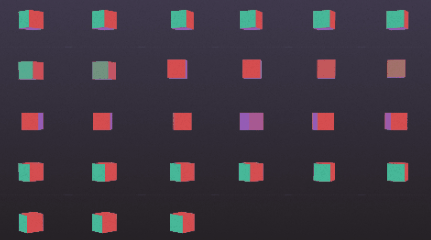Our goal is to find all the ways we can allocate the initial painting of colors to the unit cubes such that this feat is possible.

if you watched the related TED-Ed video (a portion is shown above and on the next page, with animation by Anton Trofimov) it had a method of reaching one solution, but there are more!

$($Note: We are just counting the way of allocating the colors, not different ways of placing the colors on a particular cube. For example, if a cube has $3$ red sides, $2$ green sides, and $1$ purple side, there may be multiple ways of placing those colors on a particular cube, but we're not including that in our count.$)$

# Color Cube Assembly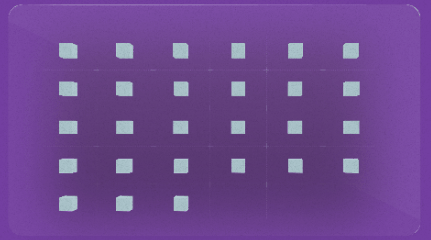When the $27$ cubes are assembled, one of the cubes fills the center, leaving $26$ cubes visible on the outside. How many "corner" cubes are there $($with $3$ sides visible$),$ how many are "edge" cubes are there $($with $2$ sides visible$)$ and how many "center" cubes are there $($with $1$ side visible$)?$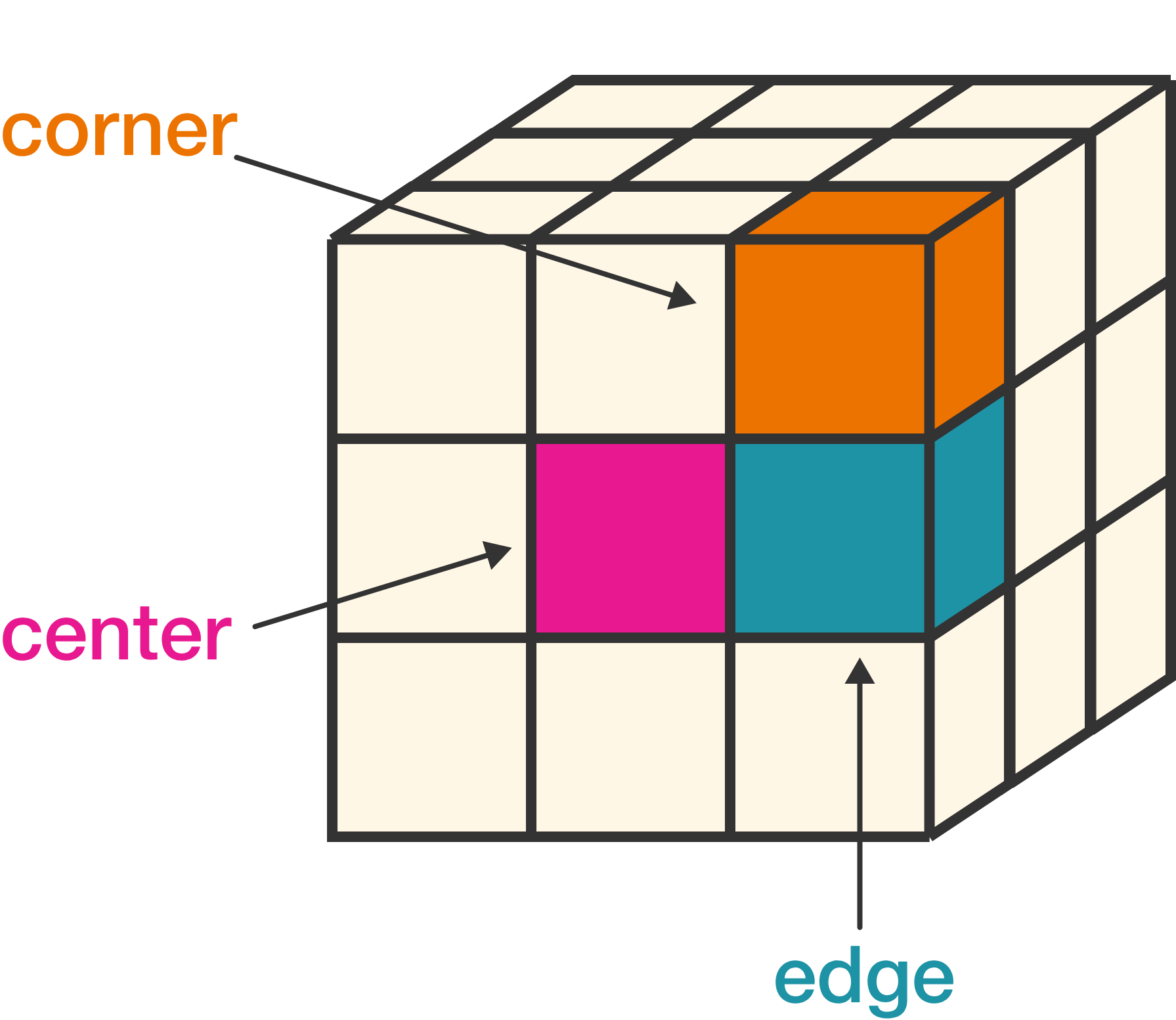# Color Cube Assembly

Remember, our goal is to be able to reassemble the $27$ cubes in three different ways so in each way only one color is visible.

Given we have $27 \times 6 = 162$ small cube faces available to paint, how many faces can we leave unpainted while still satisfying the goal of the problem?

# Color Cube Assembly

Each cube has $6$ sides, so we can think of them as adding numbers that add to 6 where each of the numbers represents a distinct color. For example, $2 + 2 + 2$ is possible and represents $2$ red sides, $2$ green sides, and $2$ purple sides.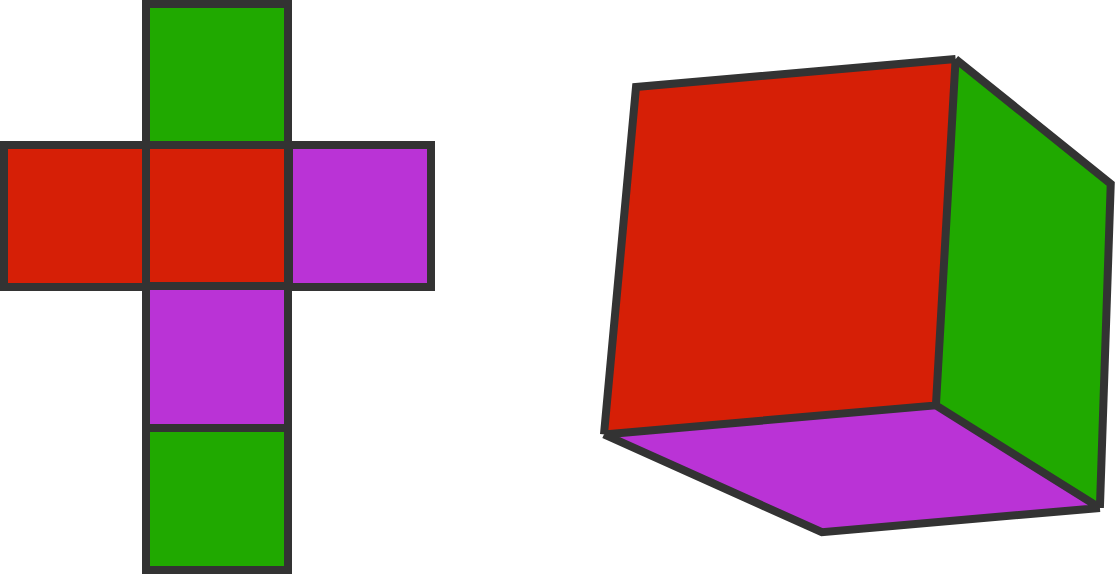What other cube types work as part of the solution? (Again, the only colors being used are red, green, and purple.)

# Color Cube Assembly

Select one or more

We've established from the previous question the cube types are $3 + 2 + 1 ,$ $2 + 2 + 2 ,$ and $3 + 3 .$ (To make it consistent with the other cube types, we will write $3 + 3 + 0$ past this point.)

Let's now count how many we'll have of each type, starting with the $3 + 2 + 1 .$ Remember this is a cube with 3 side of one color, 2 side of a second color, and 1 side of the third color.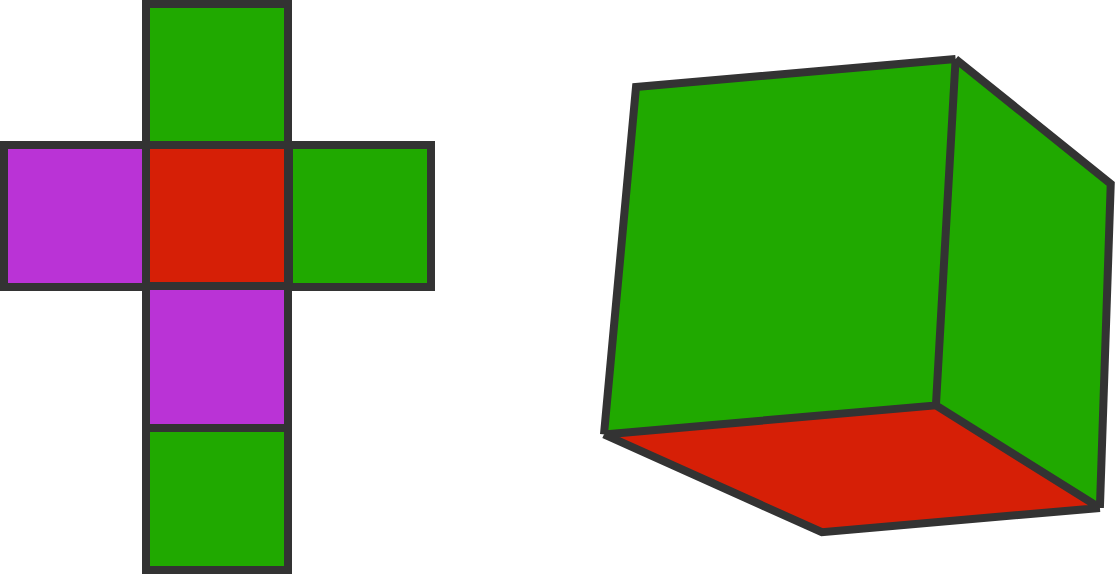How many $3 + 2 + 1$ cubes are there?

Hint: Consider how many "center" cubes you will need of each color.

# Color Cube Assembly

Continuing from the previous question, how many $3 + 3 + 0$ cubes do we have?

Note they indicate two distinct colors, but the colors can differ; one of them can be red and green while another is green and purple, for instance.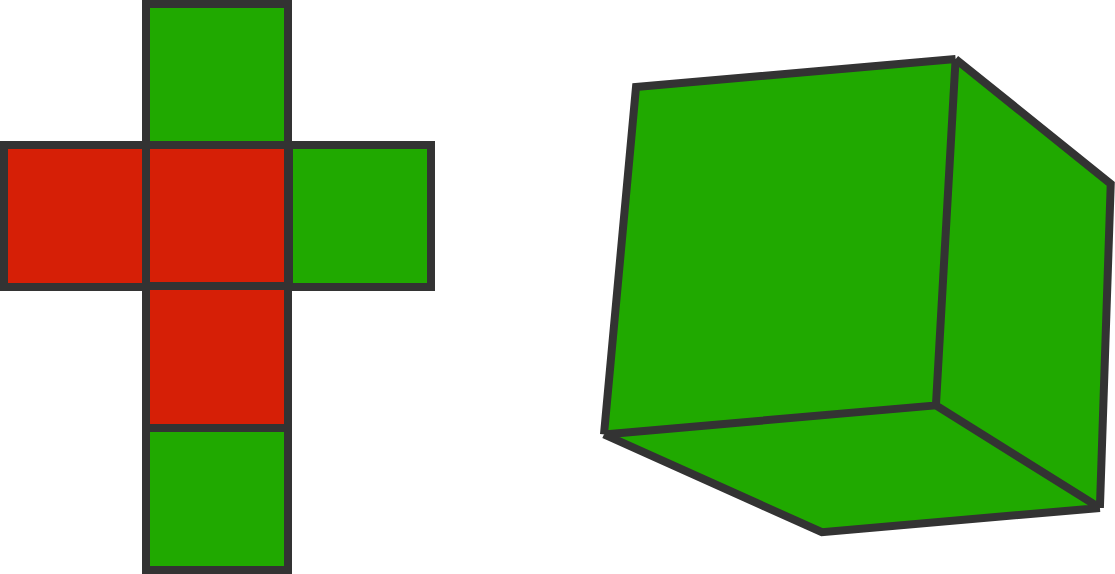# Color Cube Assembly

To summarize, we have $18$ of the $3 + 2 + 1$ cubes, and 3 of the $3 + 3 + 0$ cubes. How many $2 + 2 + 2$ cubes do we have?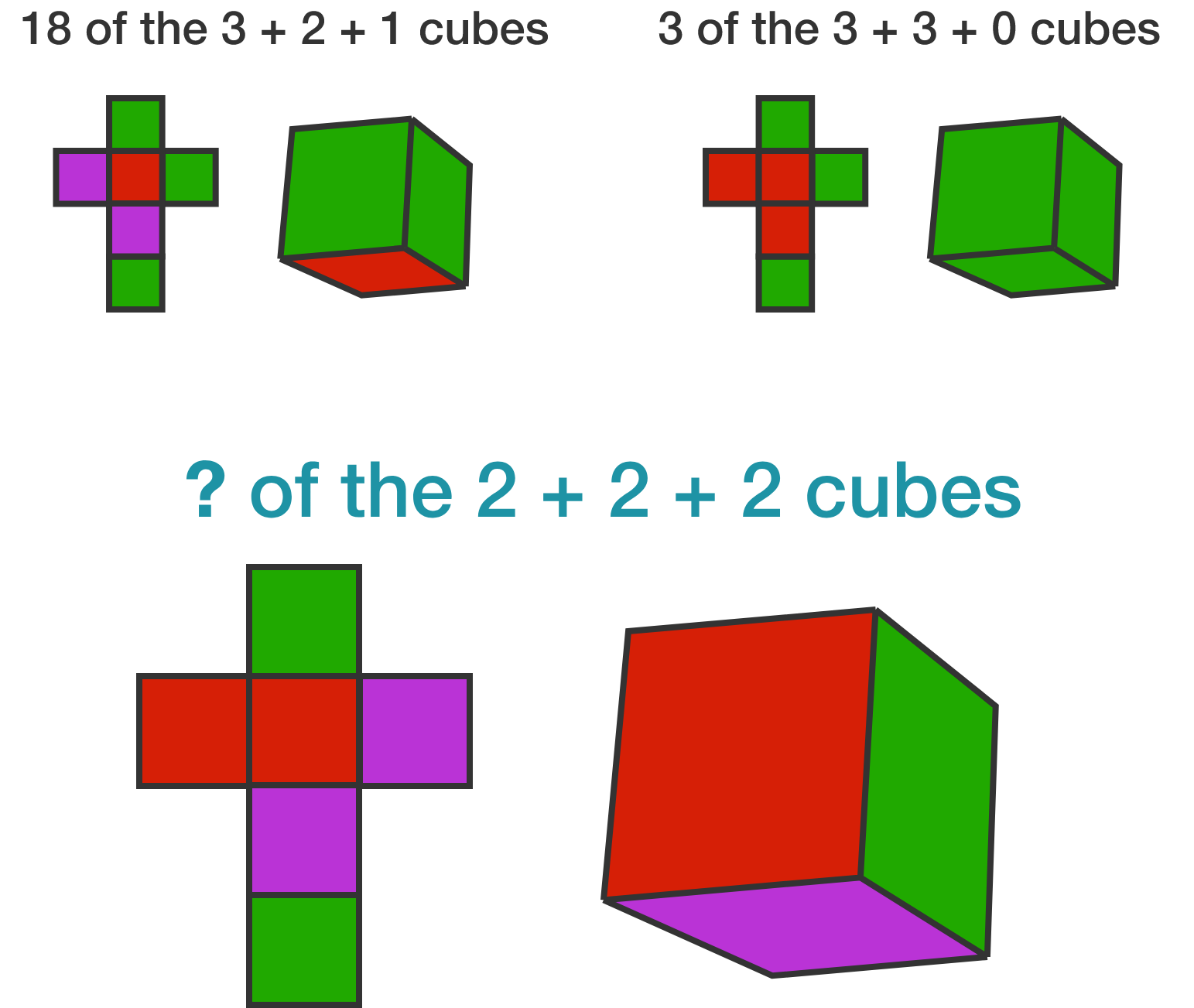# Color Cube Assembly

Note of the $2 + 2 + 2$ cubes, their color choices are already pre-set since we require all $3$ distinct colors and each one will make a $2$-face "edge" piece.There are choices for the other two types of cubes; for example, with $3 + 2 + 1 ,$ it can vary which color is a corner (matching with "$3$"), which is an edge (matching with "$2$"), and which is a center (matching with "$1$").

We'll start with the $3 + 3 + 0 ,$ where we must choose two distinct colors for each of our $3$ cubes of this type.

# Color Cube Assembly

Note that on any particular color, the six $3 + 2 + 1$ cubes are used in 6 of the corners, leaving $2$ more to be filled by the $3 + 3 + 0$ cubes.

Given this fact, which of these is a possible configuration for the $3+3+0$ cubes?

(Interpret the pairs as each giving the two colors on each of the three cubes. For example, "red/green, red/green, red/green" means that all three cubes use just the colors red and green.)

# Color Cube Assembly

We've already noted that the six $2 + 2 + 2$ cubes have fixed color configurations. The three $3 + 3 + 0$ cubes have a fixed configuration as well $($the answer in the previous question is the only way to have $2$ of each color$).$

So the only flexibility in solving the problem is setting the eighteen $3 + 2 + 1$ cubes. Here is a list of every possible way to allocate the colors: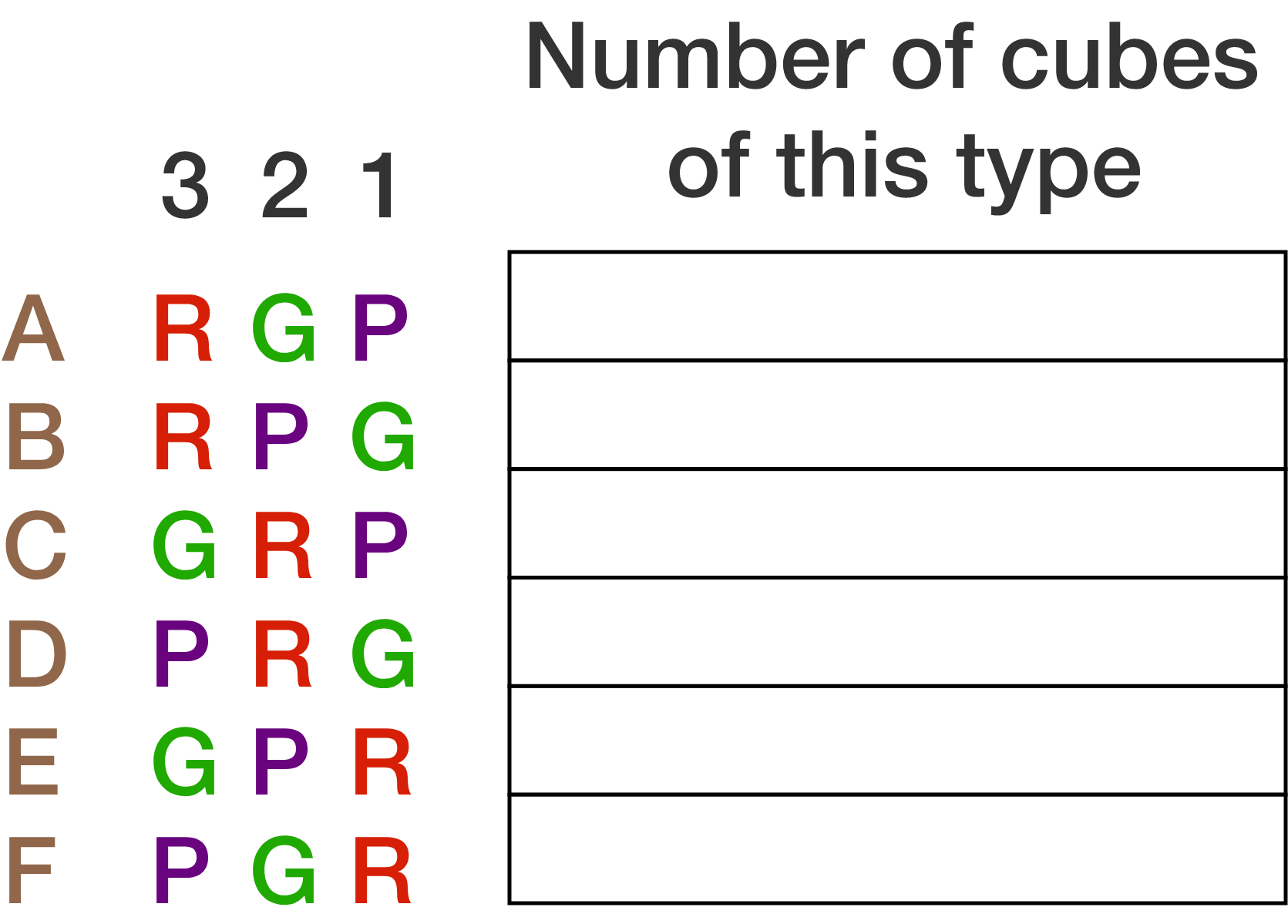(The letters A through F are added for convenience in referring to a particular cube.)

Suppose there are $2$ cubes of type A (the first row). How many cubes of type D are there?

# Color Cube Assembly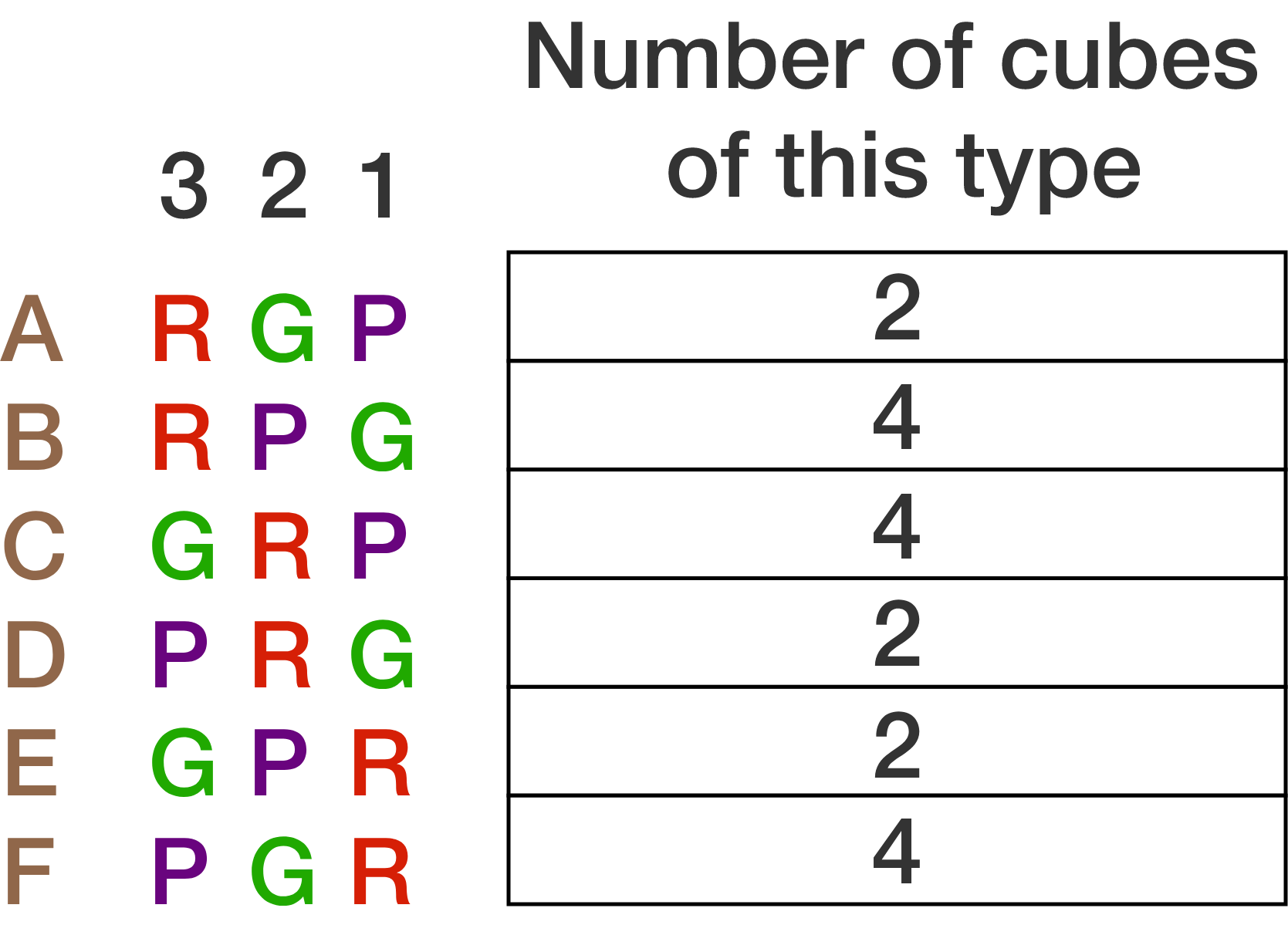Our method of finding the problem in the previous question “$2$” to fill in for cube A, but we could have filled anything from $0$ to $6.$ Since the logic after is forced, and the colors choices of the $2 + 2 + 2$ and $3 + 3 + 0$ cubes are forced, that means there are seven ways to solve the problem, each corresponding to one of the digits from $0$ to $6$.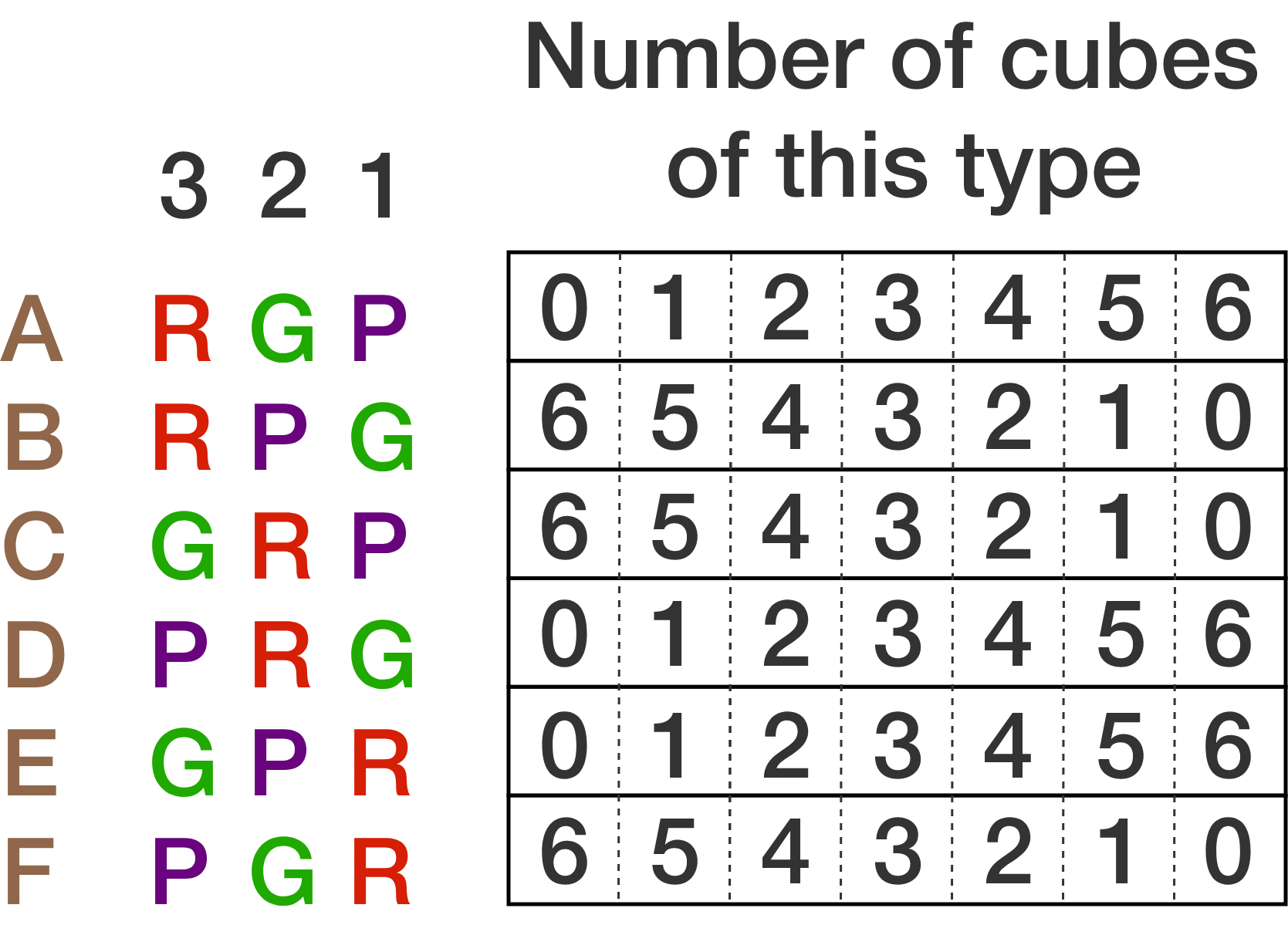If you liked the problem and want to try to extend it, here are two ways:

1. For this set of questions we just counting the way of allocating the colors, not different ways of placing the colors on a particular cube. If we were to include the physical placement on each cube in counting the number of solutions, would anything change, and if so, what?

2. What would happen if we wanted to make a $4$ by $4$ by $4$ cube instead, with $64$ small cubes total? How many colors would we be able to make? Would every side still get used in the same way as the $3$ by $3$ by $3$ case?

# Color Cube Assembly

×